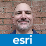# Arcpy create a polyline by csv fields

1898
2
10-12-2017 10:37 AMby
New Contributor III

Hi,

I have a csv file with different fields:

``````Address,route
First Av,[(39.72412, -104.9995), (39.72412, -105.00091), (39.72417, -105.00157), (39.72422, -105.00159)]
Second Av,[(39.72412, -104.9995), (39.72412, -105.00091), (39.72417, -105.00157), (39.72422, -105.00159)]
Street Av,[(39.72412, -104.9995), (39.72412, -105.00091), (39.72417, -105.00157), (39.72422, -105.00159)]
Street Av,[(39.72412, -104.9995), (39.72412, -105.00091), (39.72417, -105.00157), (39.72422, -105.00159)]‍‍‍‍‍``````

Whith this code i create a polyline use the array coordinate field by csv:

``````import arcpy,csv,ast
csvfile =r'lyft_1.csv'
folder=r'C:\Temp'
shapename=r'PolylineCSV.shp'
spatref_epsg=3006

icur=arcpy.da.InsertCursor(os.path.join(folder,shapename),'SHAPE@')
with open(csvfile,'rb') as f:
listrow=ast.literal_eval(row["route"])
line=arcpy.Polyline(arcpy.Array([arcpy.Point(*coords) for coords in listrow]))
icur.insertRow((line,))

del icur‍‍‍‍‍‍‍‍‍‍‍‍‍‍‍‍‍``````

But how i can insert the field address with array coordinate into Polyline?

Tags (1)
1 Solution

Accepted SolutionsbyEsri Esteemed Contributor

Note: Cross-posted to GIS Stack Exchange, but placed on on hold there as unclear.

There are several confusing aspects to this post. First off is the conflict between apparent geographic decimal degrees input (albeit in reversed order -- {Y,X} instead of {X,Y}) and the `spatref_epsg `variable (which asserts a projected coordinate system over the Caspian Sea, which is nowhere near the central Colorado implied by -105,39).  In addition, your indent scheme is wrong as posted here, since the `for `loop isn't inside the `with `which processes the input file (the answer this new question is based upon has correct indentation).  You also seem to want to use the first field in the CSV, but only insert insert the geometry field into the shapefile.  Finally, the `insertRow `request accepts a row array, but you aren't providing an array parameter.

Please update your question to have the correct Python indentation and information about the SpatialReference of the data target. It would probably help to instrument your Python code with print or arcpy.AddMessage so you can share information on the flow of control exhibited by your code.

- V

2 RepliesbyEsri Esteemed Contributor

Note: Cross-posted to GIS Stack Exchange, but placed on on hold there as unclear.

There are several confusing aspects to this post. First off is the conflict between apparent geographic decimal degrees input (albeit in reversed order -- {Y,X} instead of {X,Y}) and the `spatref_epsg `variable (which asserts a projected coordinate system over the Caspian Sea, which is nowhere near the central Colorado implied by -105,39).  In addition, your indent scheme is wrong as posted here, since the `for `loop isn't inside the `with `which processes the input file (the answer this new question is based upon has correct indentation).  You also seem to want to use the first field in the CSV, but only insert insert the geometry field into the shapefile.  Finally, the `insertRow `request accepts a row array, but you aren't providing an array parameter.

Please update your question to have the correct Python indentation and information about the SpatialReference of the data target. It would probably help to instrument your Python code with print or arcpy.AddMessage so you can share information on the flow of control exhibited by your code.

- Vby
New Contributor III

Hi Vince,

You are right! The coordinate are inverted and epsg is 4326.

I have solved my problem and this is the update code:

``````csvfile = arcpy.GetParameterAsText(0)
folder= arcpy.GetParameterAsText(1)
name = arcpy.GetParameterAsText(2)
shapename= name + ".shp"
spatref_epsg=4326
path = folder + "\\" + shapename

# Create Feature
arcpy.CreateFeatureclass_management(out_path=folder, out_name=shapename,geometry_type='POLYLINE',
spatial_reference=arcpy.SpatialReference(spatref_epsg))

icur=arcpy.da.InsertCursor(os.path.join(folder,shapename),fields)
with open(csvfile,'rb') as f: